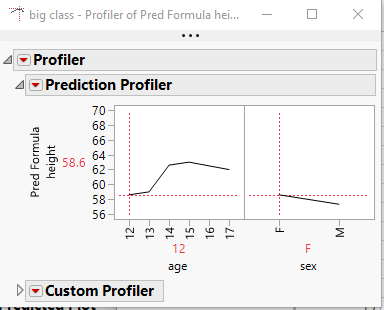Our World Statistics Day conversations have been a great reminder of how much statistics can inform our lives. Do you have an example of how statistics has made a difference in your life? Share your story with the Community!
Choose Language Hide Translation Bar
Highlighted

## How to save Contour Profiler from the Least Square Model

Hi,

How can we save only contour profiler from the least square model into separate window ?

Highlighted

## Re: How to save Contour Profiler from the Least Square Model

The way I would do it is to

1. Run the Least Squares analysis
2. Save the Predicted Formula
3. Run the Profiler Platform and point it to the saved Prediction Formula``````names default to here(1);
dt=open("\$SAMPLE_DATA/big class.jmp");

fm = dt << Fit Model(
Y( :height ),
Effects( :age, :sex, :sex * :age ),
Personality( "Standard Least Squares" ),
Emphasis( "Effect Leverage" ),
Run(
Profiler(
1,
Confidence Intervals( 1 ),
Term Value(
age( 12, Lock( 0 ), Show( 1 ) ),
sex( "F", Lock( 0 ), Show( 1 ) )
)
),
:height << {Summary of Fit( 1 ), Analysis of Variance( 1 ),
Parameter Estimates( 1 ), Lack of Fit( 0 ), Scaled Estimates( 0 ),
Plot Actual by Predicted( 1 ), Plot Regression( 0 ),
Plot Residual by Predicted( 1 ), Plot Studentized Residuals( 0 ),
Plot Effect Leverage( 1 ), Plot Residual by Normal Quantiles( 0 ),
Box Cox Y Transformation( 0 )}
)
);
fm << prediction formula;

dt << Profiler(
Y( :Pred Formula height ),
Profiler(
1,
Term Value(
age( 12, Lock( 0 ), Show( 1 ) ),
sex( "F", Lock( 0 ), Show( 1 ) )
)
),
Custom Profiler(
1,
Term Value(
age( 12, Lock( 0 ), Show( 1 ) ),
sex( "F", Lock( 0 ), Show( 1 ) )
),
Objective Formula( :Pred Formula height )
),
SendToReport( Dispatch( {}, "Custom Profiler", OutlineBox, {Close( 1 )} ) )
);``````

Jim
Article Labels